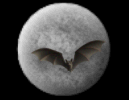God & Science Forum Message Forums: Atm · Astrophotography · Blackholes · Blackholes2 · CCD · Celestron · Domes · Education Eyepieces · Meade · Misc. · God and Science · SETI · Software · UFO · XEphemBe the first pioneers to continue the Astronomy Discussions at our new Astronomy meeting place...The Space and Astronomy Agora Probability Amplitudes? Forum List | Follow Ups | Post Message | Back to Thread Topics | In Response ToPosted by Alan on January 3, 2004 08:02:33 UTC

Idea: physics deals with real objects and imaginary numbers.

Maths deals with real numbers and imaginary objects.

"Probability" is a way of defining an object (e.g. a six sided dice is defined as 1 in 6).

"Probability amplitude" is a way of say defining a number? (e.g. square root of a nine-sided-dice is 1/3)

"Re-normalisation" is a way of not defining anything? (e.g. compare with something else and
swap places?)

Physics is supposedly involving adding "complex numbers"; known as 2-dimensional numbers.

Apparently it's the same as "ordered pairs" which would be clearer surely?

Complex number is suposed to take the form "a + ib" where i = square root of minus 1.

The "minus" is supposed to be like a vector denoting an opposite direction.

But maybe quantum electro-dynamics can be seen in as a game with numbers?

The probability of getting one face of six facing upwards in tossing a dice is 1 in 6. That is just a definition of a tossed dice: 1 in 6 defines it.

In quantum physics they take "the square root of a probability" add it to other such "probability amplitudes" to get a final probability amplitude (Done by adding little arrows on a piece of paper head to tail in any order, then taking a final arrow from start of first arrow to end of last arrow. They square the final arrow and say this is the probability that an event will happen. But does it involve circular reasoning?)

(For an event that happens in steps they "turn and shrink the arrow against a unit arrow).

By the way: this seems to work: the distributive law says 3 (a + b + c) = 3a + 3b + 3c. What if I replace "3" with "z", and allow all these potential variables to swap places?

With numbers fixed you cannot do that in math as
3 (a + b + c) doesn't equal a (3 + b + c).

3 (1 + 2 + 3) = (3x1) + (3x2) + (3x3) = 18.
1 (3 + 2 + 3) = (1x3) + (1x2) + (1x3) = 8.

This is supposed to be unequal as 18 doesn't equal 8 according to math. But math assumes that numbers are all equally spaced and equal sized units.

But numbers are just stickers or labels stuck to things? The things themselves are not equal: they must be different to be countable or you can only count one item.

Richard Feynman mentioned in his book "QED. The Strange Theory Of Light And Matter" about "the math being too hard for us". He also mentioned a "dippy process" called "renormalisation" that doesn't seem to be mathematical.

Suppose "the math is too hard" because it makes numbers into rigid units of equal size. But how is number defined? Seems that when you divide a total in half; you don't say which half is bigger, you just say it is two pieces.

Divide it somewhere else and it could be pretty unequal shares? But math potentially goes "back in time" and could shift the place of the first break to define it and the new break as equal spaced breaks?

The parables in the Gospels in the Bible seem to tell us a lot about how "number" is seen in the Kingdom of Heaven.

Maybe physics is "what is missing in mathematics"; physics laws might show up the assumptions in a fixed mathematics view of number say?

But what if I do not use numbers and use letters?

Maybe that allows the issue of "a fixed grid of equal units" to stay "indeterminate" until everything has been considered in the scenario being looked at.

In QED they say the length of the arrow is "the square root of a probability" and the direction is where the hand on a stop-watch points after going around many times between start and finish.
They say for different wavelengths of light this "stopwatch" goes around a different number of times per second.

If I look at the distributive law and use all letters:

I get z (a + b + c) = za + zb + zc.
If I break the rules of rigid math and suggest "z" can swap places with a, with b, with c; I get a perspective that might model how QED works.

The direction of the arrow would be the "z" role; the length of the arrow would be the (a + b + c) role.

To know these roles are different would require comparing with some other problem called: "renormalisation".

"mass" of ideal electron would be "z"; "charge" of ideal electron would be "a + b + c".

If the length of the arrow is "square root of a probability" and if "square root of a probability" just means "square root of a ratio of numbers that defines something like a one dice with six faces"; then square root of a fixed ratio would be a way of looking at it so that it could (in the dice example) be 6 views of 1 OR 1 view of 6 OR take another perspective into this also?

Maybe allowing "z" to swap with a, b, and c makes probability "a + b + c" into square root of probability? Maybe allowing "a, b and c" to swap places with "z" allows them to give direction to "z"?

-dolphin